Courses
Courses for Kids
Free study material
Free LIVE classes
More

# JEE Important Chapter - Mechanics

Get plus subscription and access unlimited live and recorded courses## Mechanics for JEE

Last updated date: 01st Jun 2023
Total views: 115.2k
Views today: 3.13k

Mechanics is a branch of physics that deals with energy and forces and their effect on bodies. As far as JEE Advanced exam is concerned, this chapter covers a vast amount of topics and is thus a very important chapter. This chapter requires conceptual understanding as well as numerical skills to score good marks in JEE Advanced.

In Mechanics, we learn about one dimensional and two dimensional and practical applications of newton’s laws of motion and force system in mechanics. Concepts related to kinetic energy and potential energy are also discussed in detail along with the work-energy theorem.

Problems related to rotational dynamics and gravitation are also important in Mechanics. Concepts related to the properties of solids and liquids as well as thermodynamics are included in this chapter mechanics.

Now, let us see what is mechanics, mechanics definitions, laws of mechanics, important concepts and formulae related to JEE advanced exams along with a few solved examples.

### Important Topics of Mechanics

• Motion along two dimension

• Laws of motion

• Mass Pulley system

• Work done by a constant force and variable force

• Kepler’s Law of planetary motion

• Stress-strain curve

• Rotational motion

• Bernoulli’s theorem

• Laws of thermodynamics

### Important Concepts of Mechanics

 Name of the Concept Key Points of Concept 1. Kinematic equations in one-dimensional motion Equations of motions for uniformly accelerated motion is given by,$v=u+at$$s=ut+\dfrac{1}{2}at^{2}$$v^2=u^2+2as$Where u is the initial velocity, v is the final velocity, a is the acceleration and t is the time taken.The instantaneous velocity and instantaneous acceleration of a body is given by the formula,$v=\dfrac{dx}{dt}$$a=\dfrac{dv}{dt}=\dfrac{d^2x}{dt^2}For motion under gravity, take acceleration as 9.8 m/s2. While applying equations of motion in motion under gravity, take one direction positive and the opposite direction. For eg: If you take upward direction as positive, then take upward velocity as positive and acceleration due to gravity in the downward direction negative. 2. Graphs for one-dimensional motion Displacement-time graphThe slope of a displacement time graph gives velocity.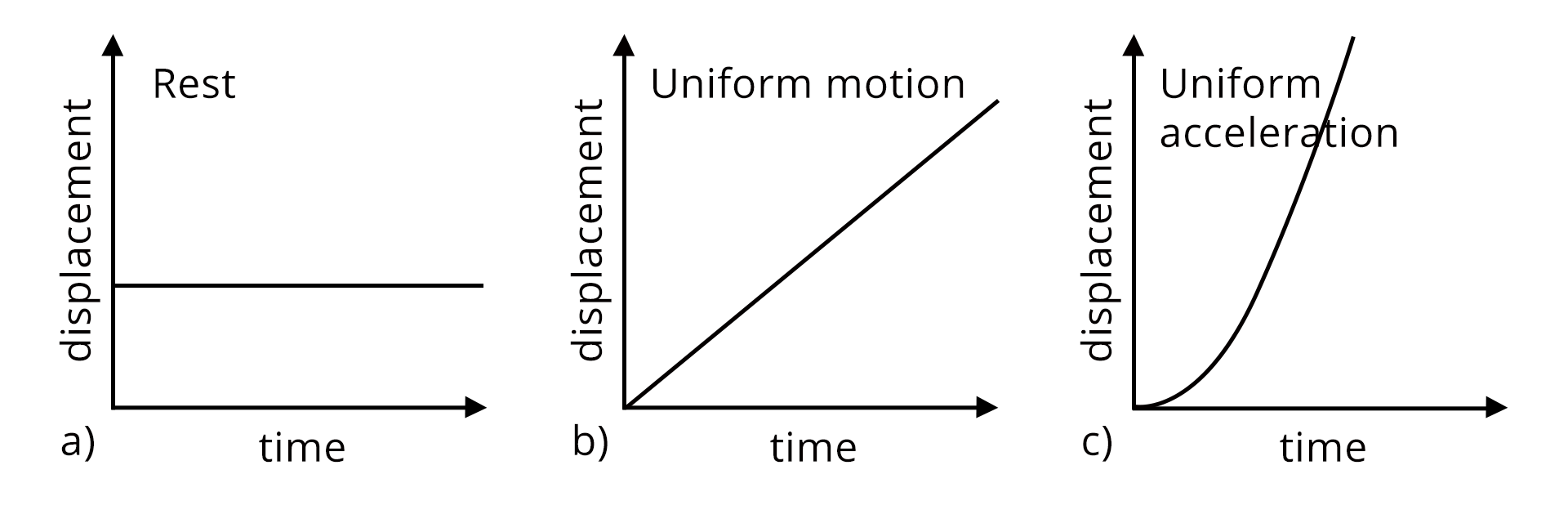Velocity-time graphThe slope of a velocity-time graph gives the displacement covered by the body.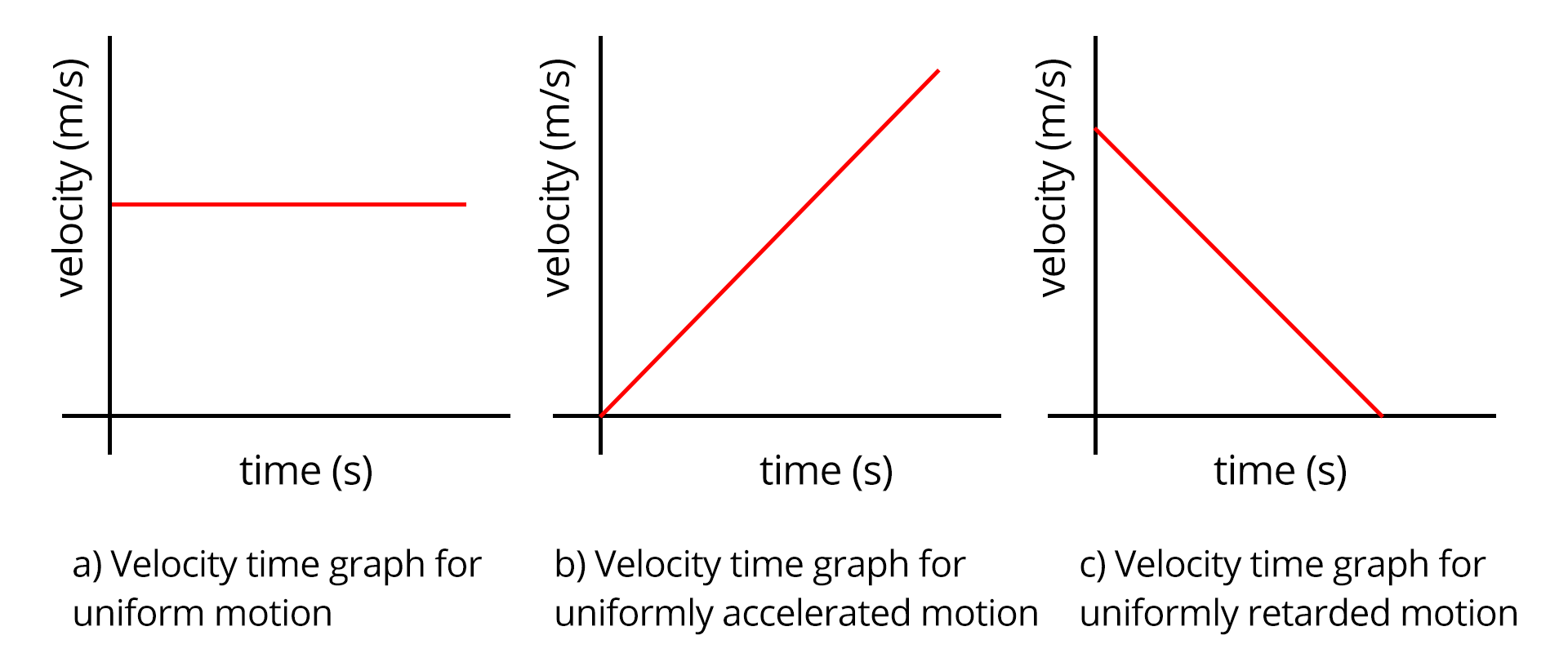Acceleration-time graphThe area under the curve of the acceleration time graph gives the change in velocity.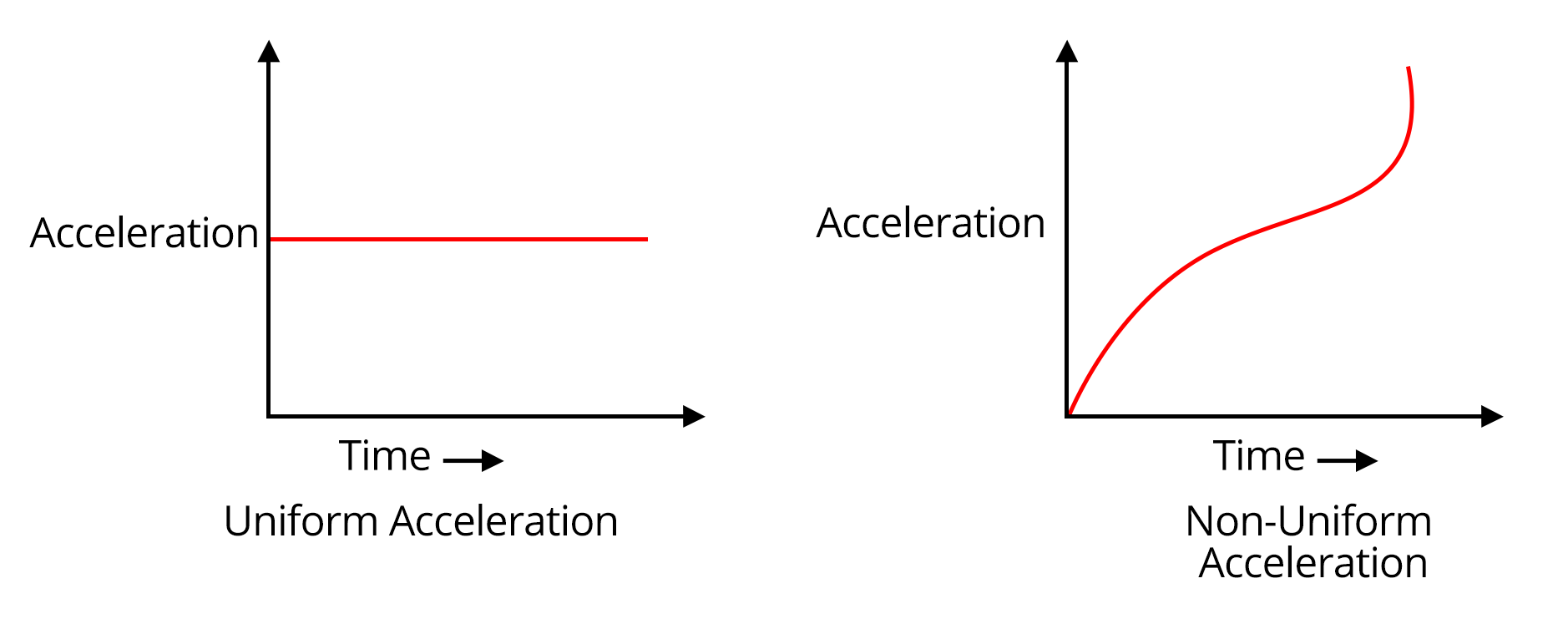3. Motion along two-dimension When the motion of a body is along a plane, it is called two-dimensional motion. Examples are Projectile motion and circular motion.In a projectile motion, the body moves under the influence of gravity.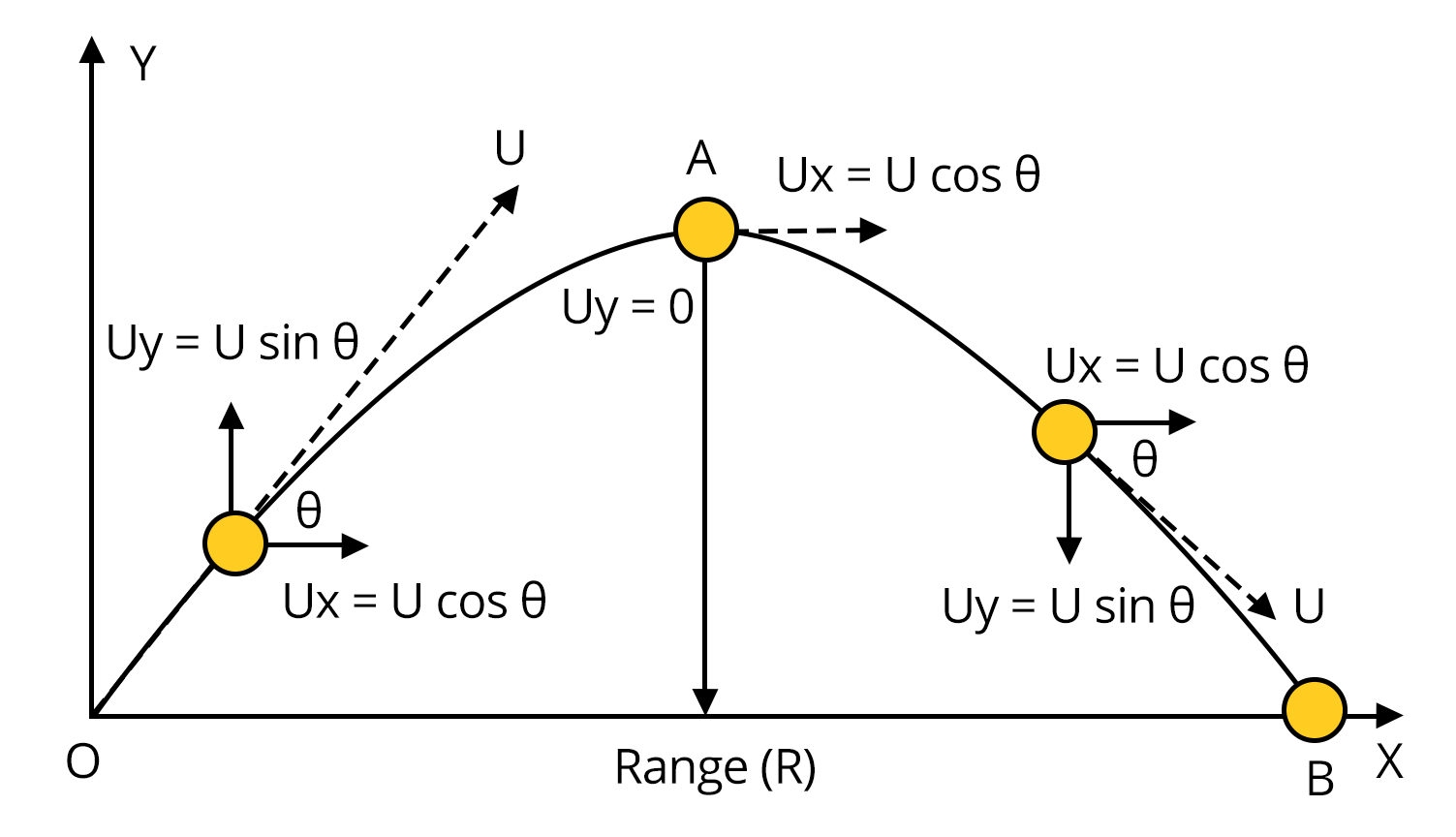The range of the projectile motion is given by,R=\dfrac{u^2\sin (2\theta)}{g}Time of flight is the total time taken by the body to reach back to the ground.T=\dfrac{2u\sin (\theta)}{g}Maximum height of the projectile motion is given by,R=\dfrac{u^2\sin^2 (\theta)}{2g}Where u is the velocity at which the body is projected, θ is the angle of projection and g is the acceleration due to gravity. In a uniform circular motion, speed remains constant.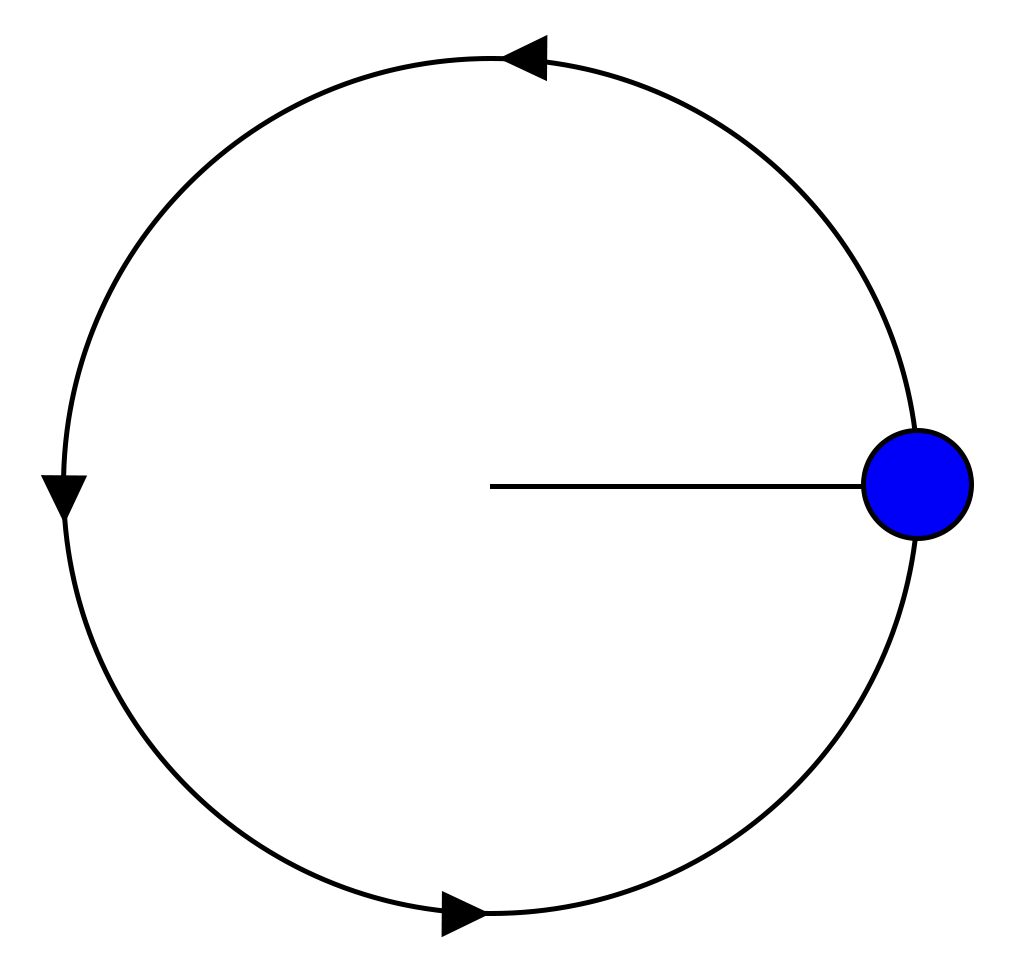The relation between linear velocity (v) and angular velocity (⍵) of a particle moving in a circle of radius r is,v=r\omegaAngular acceleration is the rate of change of angular velocity with respect to time.\alpha=\dfrac{d\omega}{dt}=r\omega^2The relationship between linear acceleration (a) and angular acceleration (α) isa=r\alpha 4. Laws of Motion Newton’s first laws of motion state that a body continues to be in a state of rest or state of uniform motion along a straight line until and unless an external unbalanced force is acting on it.According to newton’s second law, the net force acting on a body is equal to the rate of change of momentum.\vec F=\dfrac{d\vec P}{dt}=m\vec aNewton’s third law of motion states that every action has an equal and opposite reaction.While dealing with problems related to laws of motion, always draw the free body diagram and write equations using the laws of motion of the free body mechanism. Law of Conservation of momentum: The total momentum of a system is conserved if the external force acting on the system is zeroFriction: It is the force that opposes the relative motion between two surface in contact.Friction is independent of area of contact and depends on the normal force acting between the two surface.The formula for static friction and kinetic friction is given belowf_{s}=\mu_sN$$f_{k}=\mu_kN$Static friction is a self adjusting force 5. Mass Pulley system For a mass pulley system as shown below, the magnitude acceleration for both blocks will be same.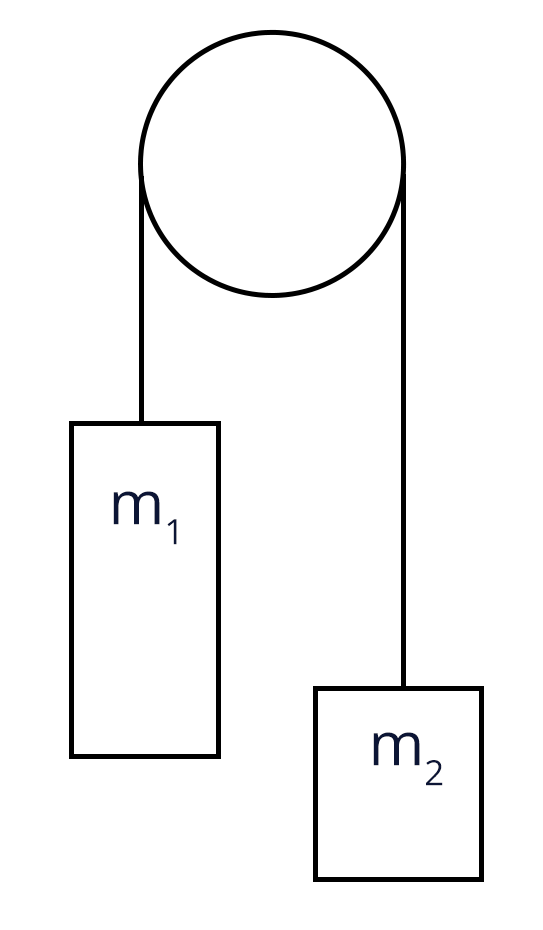Tension acting on the string is given by,$T=\dfrac{2m_1m_2g}{m_1+m_2}$The acceleration of each block is given by,$a=\dfrac{(m_1-m_2)g}{m_1+m_2}$ 6. Work done by a constant force and variable force Work is a scalar quantity and the work done by a constant force is given by,$W=\vec F\cdot \vec d$$W= Fd\cos\theta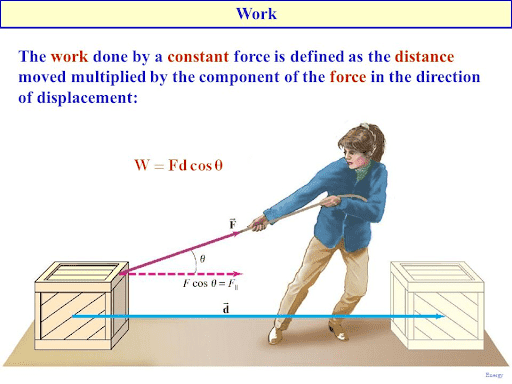Work done by a variable force is given by,W=\int FdsThe area under the curve of force-displacement graph also gives the work done by the force.Work- Energy theorem: It states that work done by net force on a body is equal to change in kinetic energy. 7. Rotational motion Parallel axis theorem: The theorem of parallel axis states that the moment of inertia of a body about an axis parallel to an axis passing through the centre of mass is equal to the sum of the moment of inertia of body about an axis passing through centre of mass and product of mass and square of the distance between the two axes.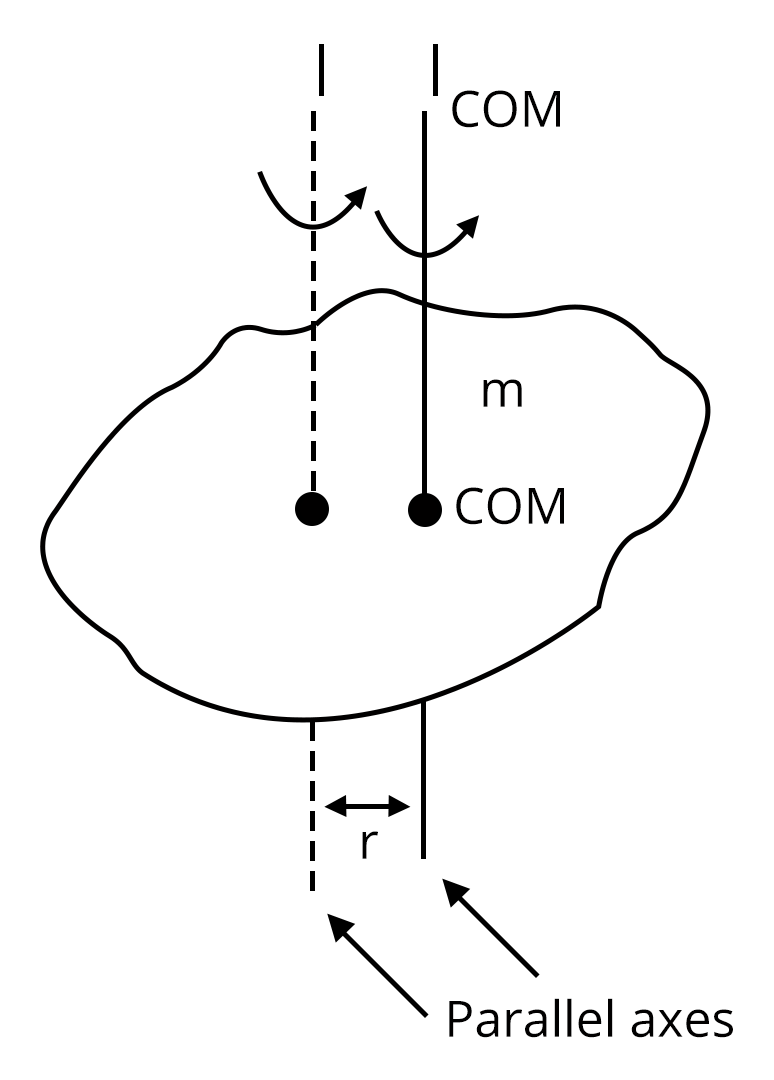I=I_{COM}+Mr^2Perpendicular axis theorem: This theorem states that the moment of inertia of a planar body about an axis perpendicular to its plane is equal to the sum of its moments of inertia about two perpendicular axes concurrent with the perpendicular axis and lying in the plane of the body.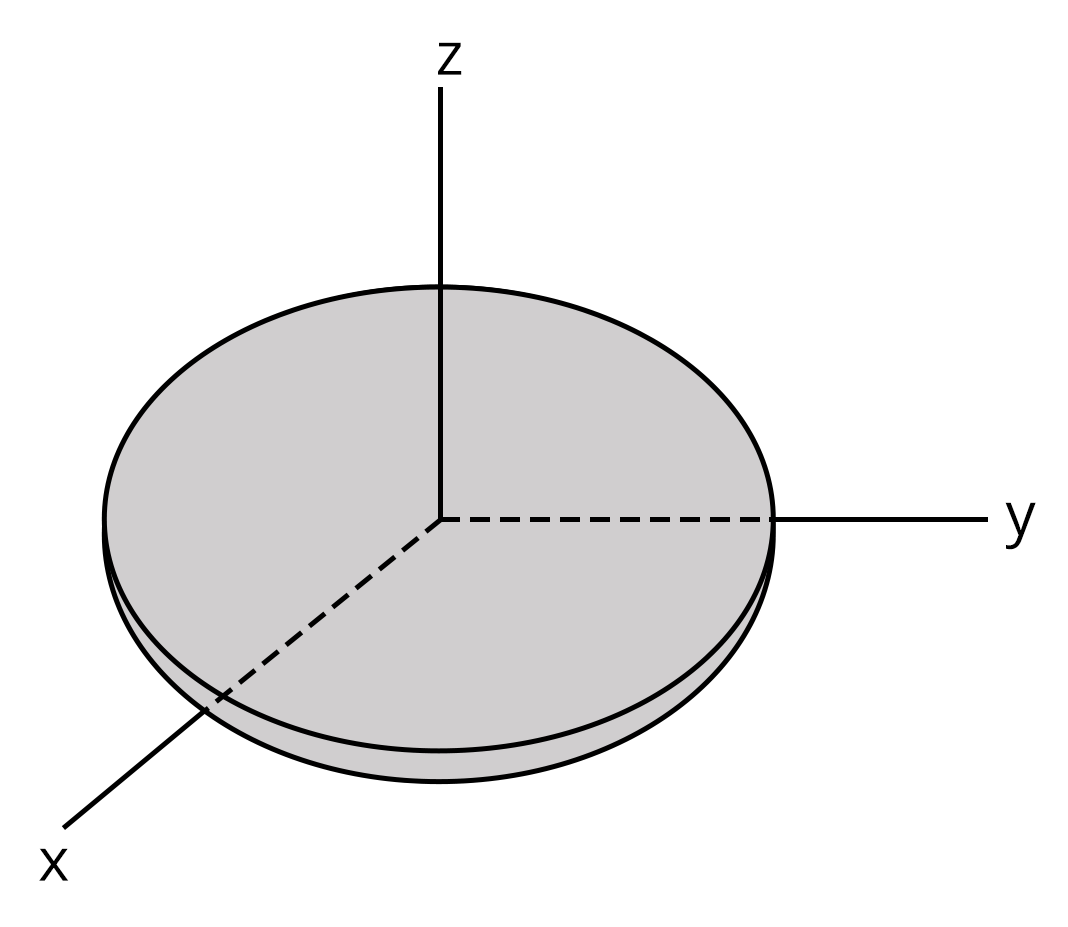I_z=I_{x}+I_yThe torque required to rotate a body having moment of inertia(I) with an angular acceleration(⍺) is given by,\tau=I\alphaThe anglular momentum of a rotating body is given by, L=I\omegaThe condition for a body to be in pure rolling motion is given by,v_{cm}=R\omega 8. Gravitation The gravitational force acting between two bodies m_1 and m_2 is given byF=\dfrac{Gm_1m_2}{r^2}Acceleration due to Gravity of Earth below the Earth’s Surface is given by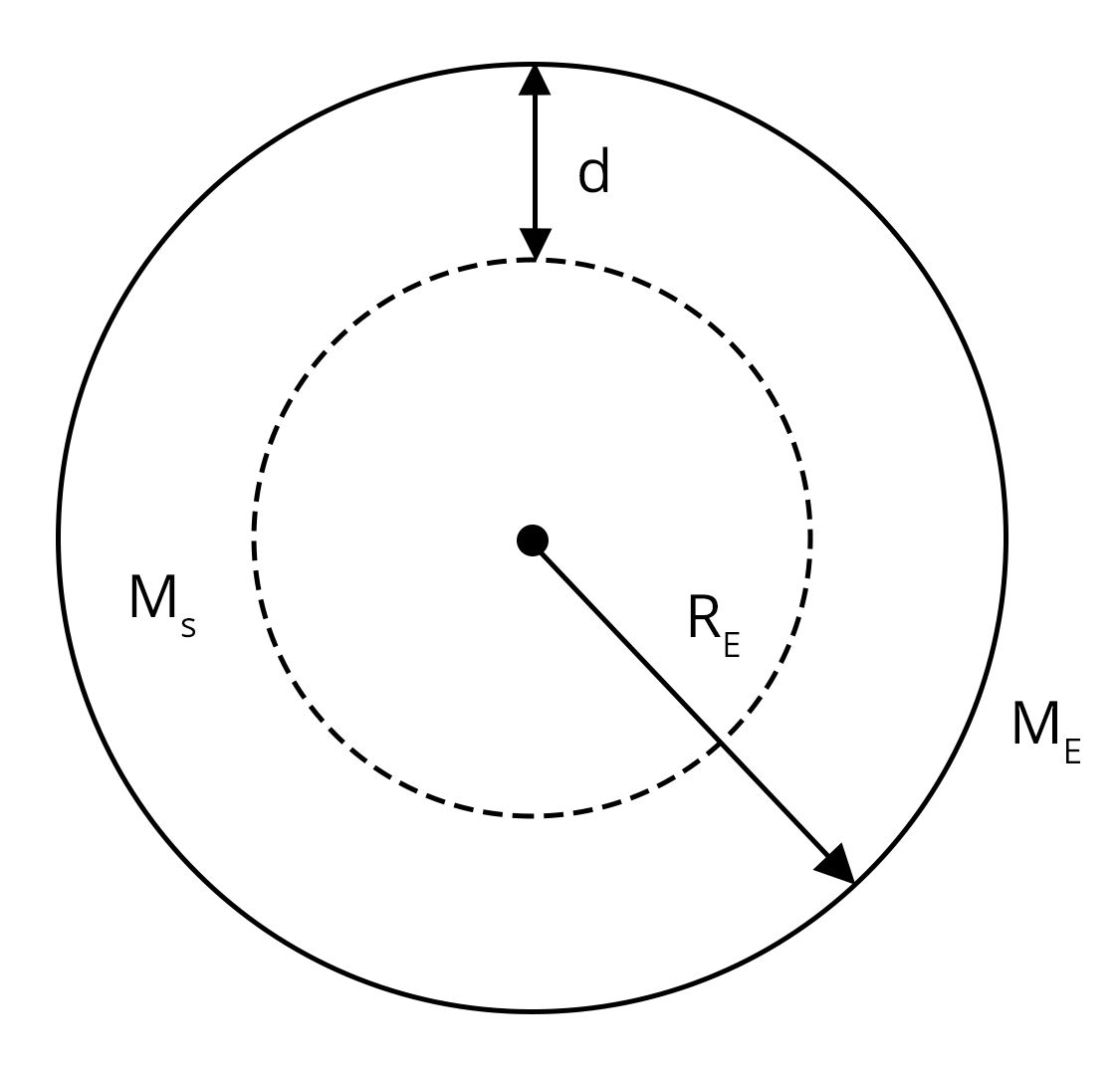g'=g\left(\dfrac{R_E-d}{R_E}\right)Acceleration due to Gravity of Earth above the Earth’s Surface is given by,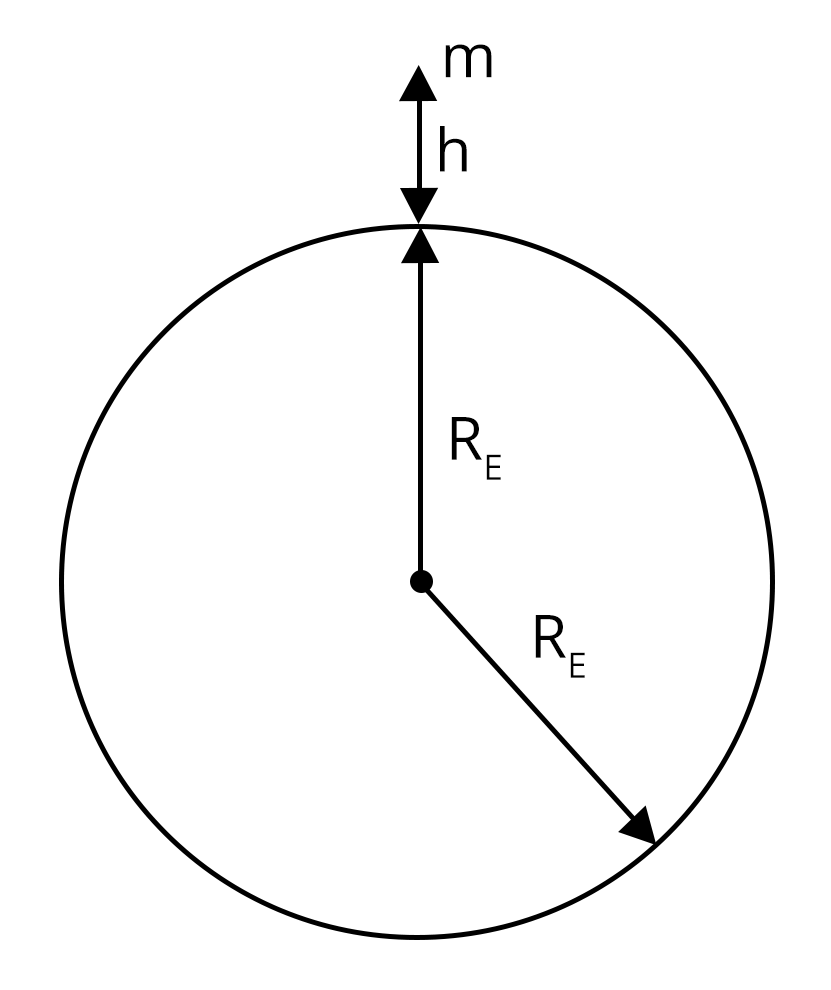g'=g\left(1-\dfrac{2h}{R_E}\right)The gravitational potential energy between two masses is given by,U=-\dfrac{Gm_1m_2}{r}Escape velocity is the minimum velocity required for a body to escape from the gravitational field of the earth.v=\sqrt{2gR_E} 9. Kepler’s Law of planetary motion Kepler's Law consists of three laws.Law of Orbits: All planets move in elliptical orbits with the Sun situated at one of the foci of the ellipse.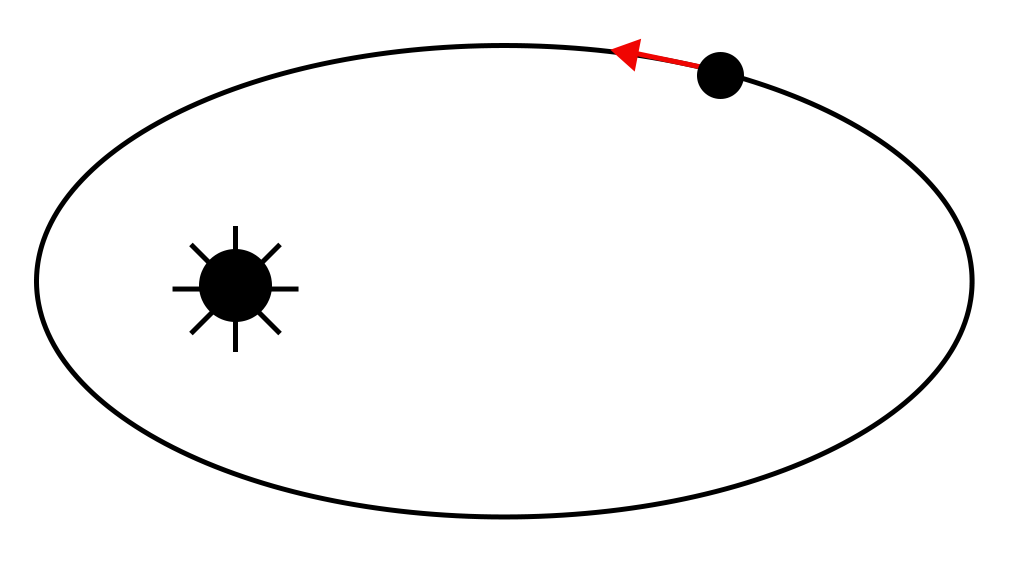Law of Areas: The line that joins any planet to the sun sweeps equal areas in equal intervals of time.Law of Periods: The square of the time period of revolution of a planet is proportional to the cube of the semi-major axis of the ellipse traced out by the planet.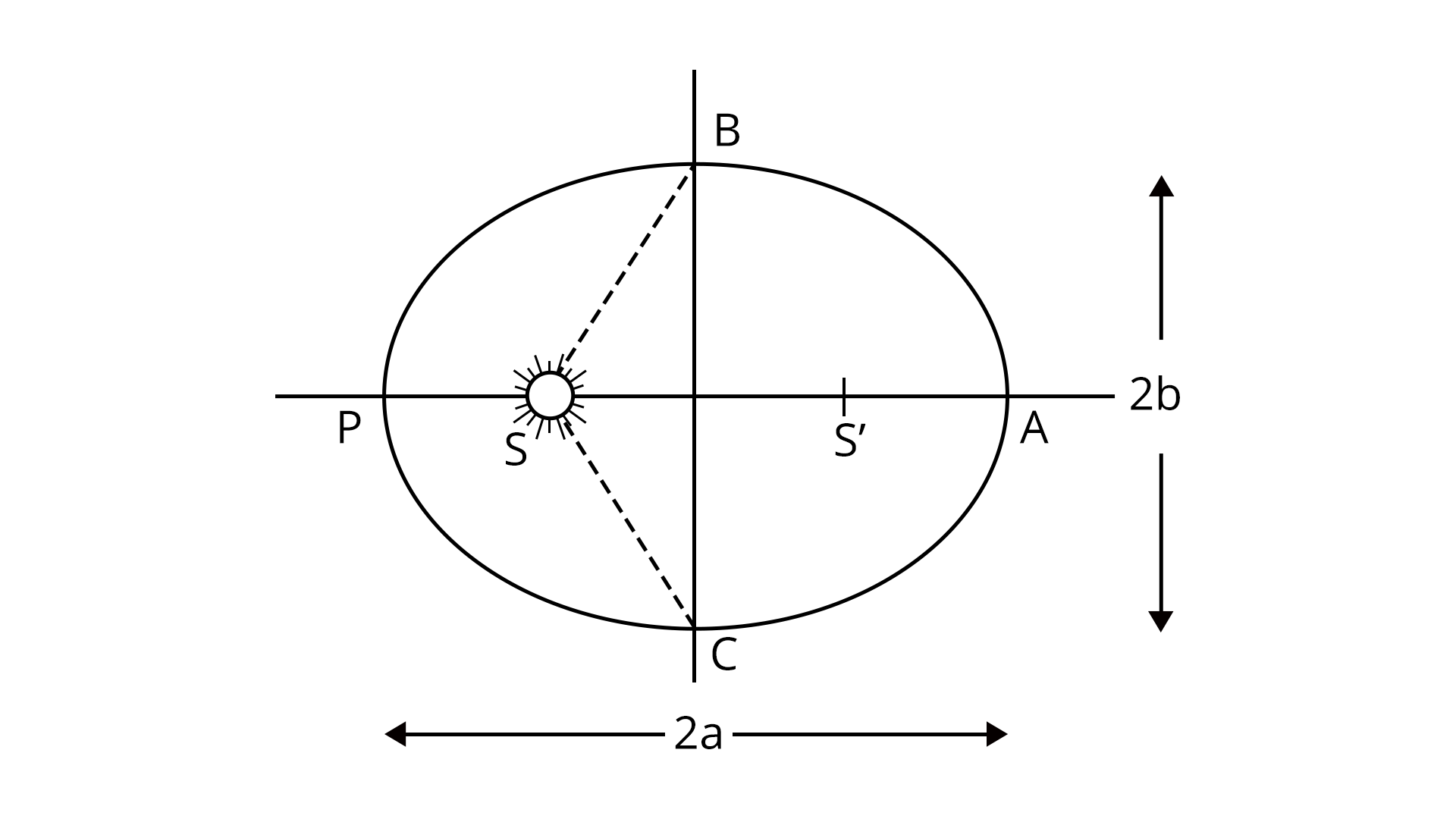10. Stress-strain curve The behaviour of a material can be studied using stress-strain curve.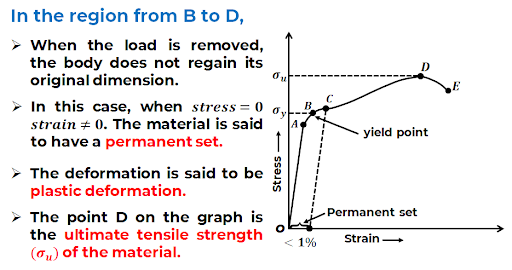The curve obeys Hooke’s law in the region from O to A. According to Hookes law. Stress is proportional to strain.In the stress-strain curve given above, the point A corresponds to proportional limit. The yield point or elastic limit is the point upto which the wire shows elastic behaviour and the point B corresponds to the yield point.The stress corresponding to the yield point is called yield strength.Beyond point B, it loses its elastic behaviour and does not regain its original length even after deforming force is removed.The stress corresponding to point D is called ultimate strength. 11. Archimedes principle According to Archimedes principle, whenever a body is fully or partially submerged in liquid, it experiences a net upward force which is equal to the weight of the liquid displaced.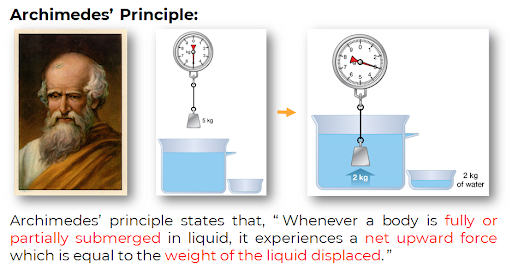The formula to calculate the buoyancy force is given by,F_B=\rho_l VgWhere ⍴l is the density of the liquid, V is the volume of the body immersed in the liquid and g is the acceleration due to gravity.A body will float in a liquid if the buoyancy force acting on the body is equal to the weight of the body. 12. Bernoulli’s theorem According to Bernoulli’s theorem, the total energy per unit volume of an incompressible fluid always remains constant at any point of the fluid.P+\rho gh+\dfrac{1}{2}\rho v^2=\text{constant}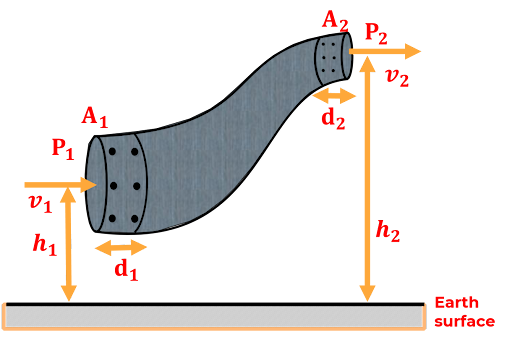P_1+\rho gh_1+\dfrac{1}{2}\rho v_1^2=P_2+\rho gh_2+\dfrac{1}{2}\rho v_2^2 13. Laws of thermodynamics It states that heat given to a system(ΔQ) is equal to the change in internal energy(ΔU) and work done by the system(ΔW).\Delta Q=\Delta U+\Delta W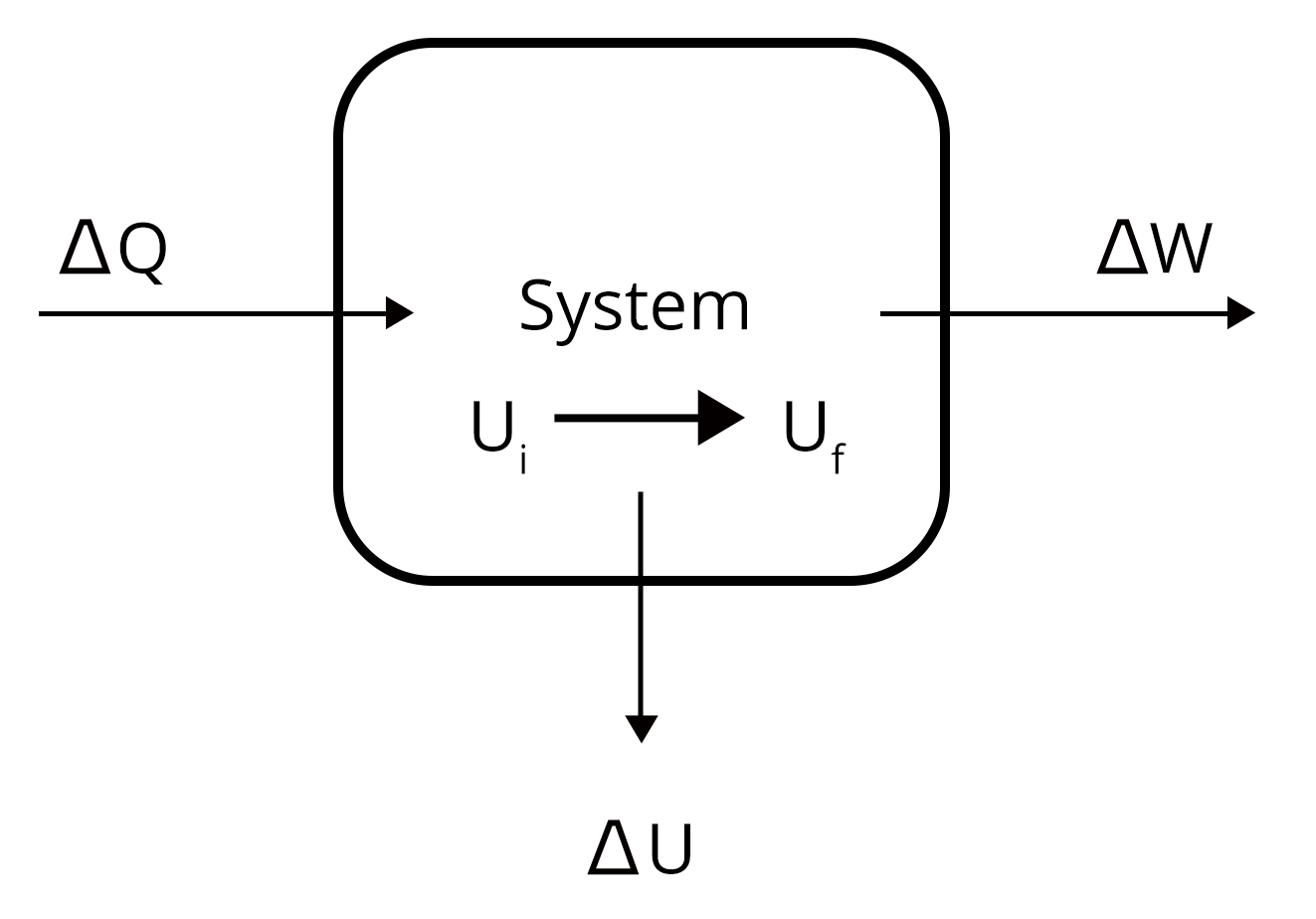Second law of thermodynamics:Clausius statement: It is not possible to construct a device that operates on a cycle and produces no other effect than transferring heat from a colder body to a hotter body Kelvin- Plancks statement: It is impossible to design an engine operating in cycles that absorb heat and deliver the equivalent amount of work. 14. Carnot engine and refrigerator Carnot engine is an ideal heat engine designed by french engineer Carnot.The working substance in the Carnot engine undergoes a cyclic process called carnot cycle.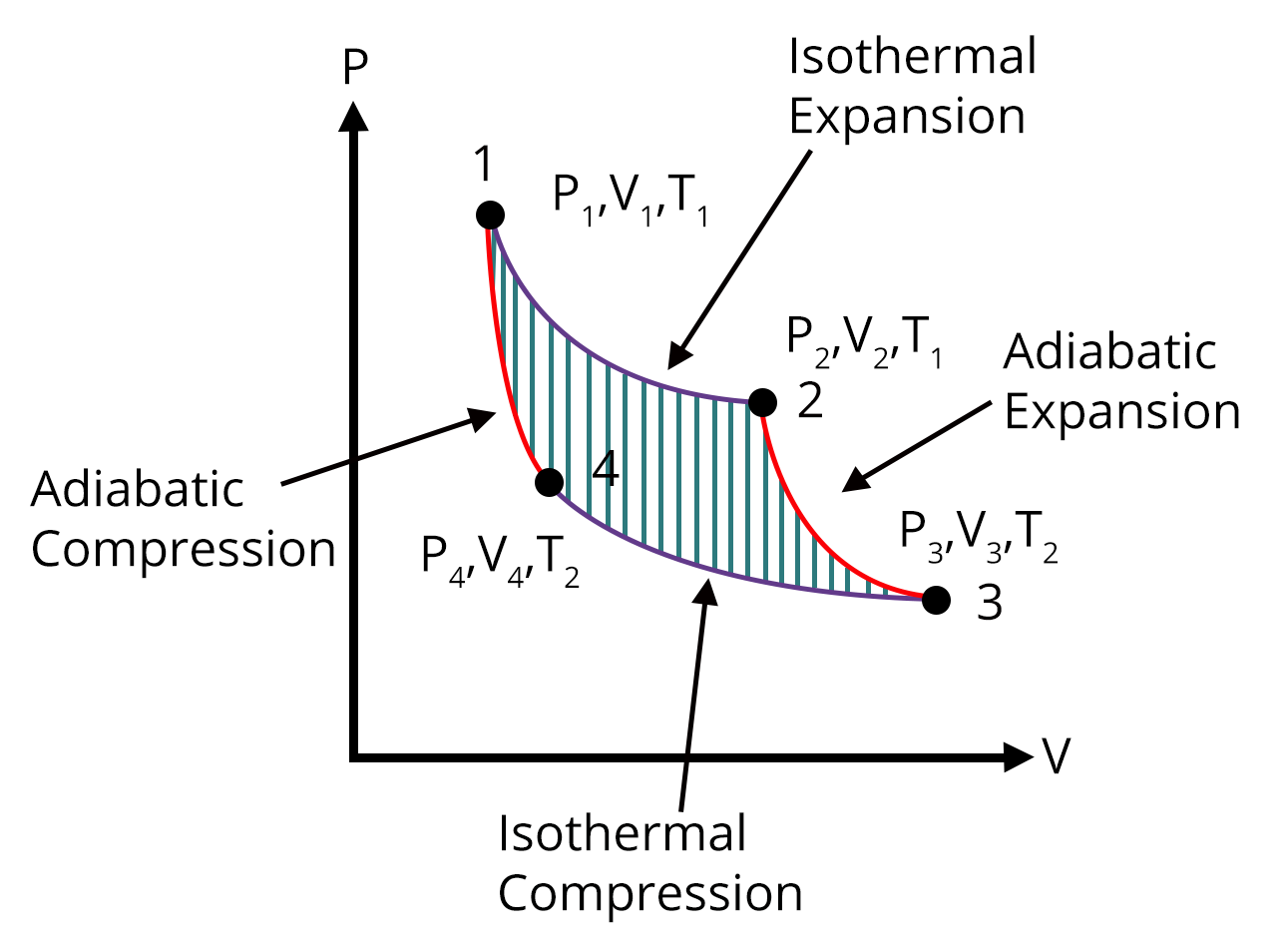Area of the P-V graph of carnot cycle gives the work done during one cycle.The efficiency of the carnot cycle is given by,\eta=\dfrac{T_1-T_2}{T_1}A refrigerator is a device that transfers heat energy from colder body to a hotter body by doing some external work on it.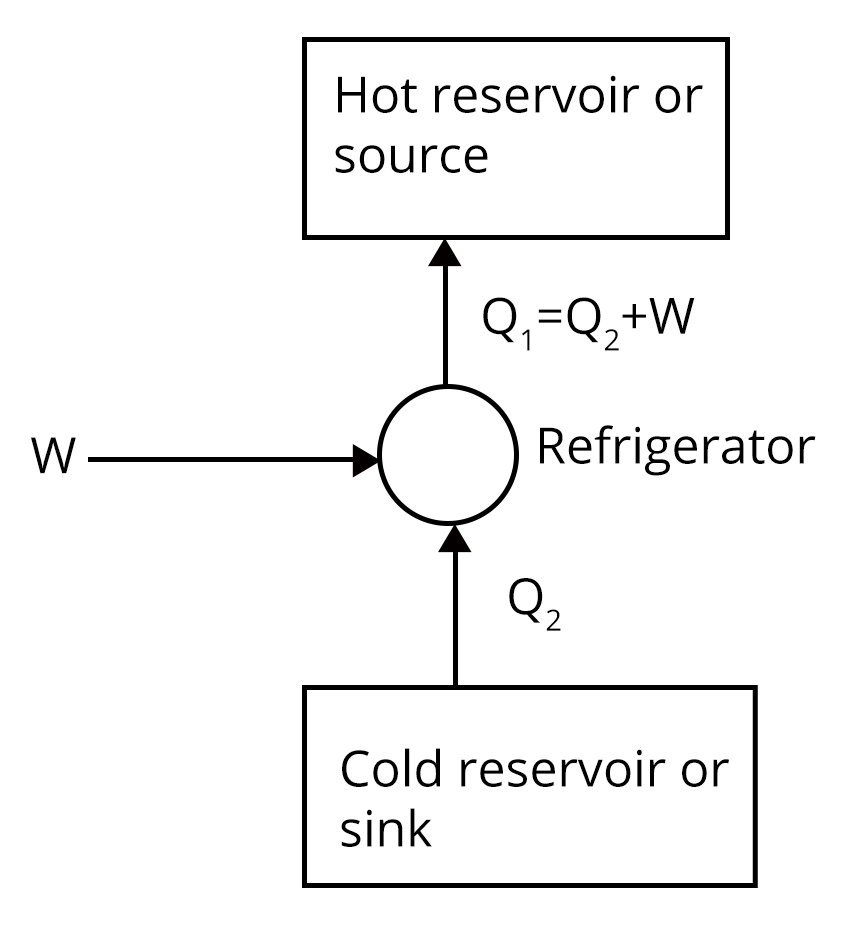The coefficient of performance of a refrigerator (C.O.P) is given by,\beta=\dfrac{Q_2}{W}$$\beta=\dfrac{Q_2}{Q_1-Q_2}$

### List of Important Formulae

 Si. No Name of the Concept Formula 1. Addition and subtraction of two vectors $|\vec A +\vec B|=\sqrt{A^2+B^2+2AB\cos\theta}$$|\vec A -\vec B|=\sqrt{A^2+B^2-2AB\cos\theta}$Where A and B are the magnitude of the two vectors at an angle θ. 2. The motion of a body projected from the top of a tower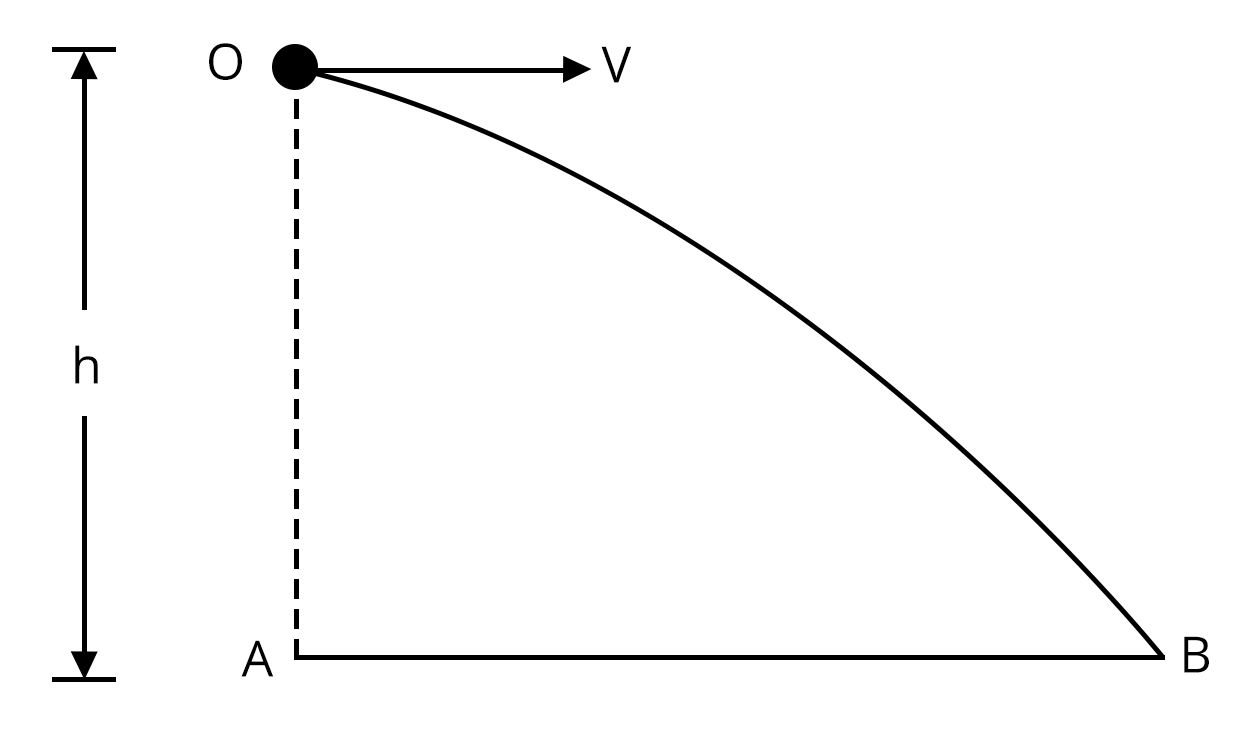The trajectory of the body is given as$y=\dfrac{gx^2}{2u^2}$Where y is the vertical displacement and x is the horizontal displacement. 3. The average velocity of the body in a journey that covers two equal distances with two different speeds $v_{avg}=\dfrac{2uv}{u+v}$Where u and v are the two different speeds by which the body covers two equal distances. 4. The displacement covered in nth second of a uniformly accelerated body $S_{n}=u+\dfrac{1}{2}a(2n-1)$Where u is the initial velocity, a is the acceleration and Sn is the displacement covered by the body in the nth second. 5. Maximum safe speed for a a car moving in a circular track $v_{max}=\sqrt{\mu Rg}$ 6. Potential energy stored in a stretched string $U=\dfrac{1}{2}kx^2$ 7. Moment of inertia of a disk $I=\dfrac{MR^2}{2}$ 8. Moment of inertia of a ring $I=MR^2$ 9. Moment of inertia of a solid sphere $I=\dfrac{2}{3}MR^2$ 10. Work done in stretching a strain Work done in stretching a wire having young’s modulus(Y) under a force (F) is given by the formula,$W=\dfrac{1}{2}\dfrac{YA\Delta l^2}{l}$ 11. Thermal stress on a rod fixed between two rigid supports. $\text{Thermal stress }=Y\alpha\Delta T$Where ⍺ is the coefficient of linear expansion and ΔT is the change in temperature 12. Equation of continuity $A_1V_1=A_2V_2$Where A1 and A2 are the area of cross section of the pipe at the two ends and V1 and V2 are the corresponding velocity of the fluid.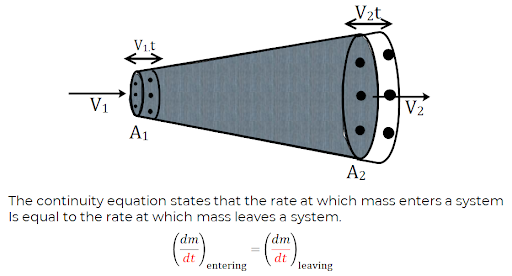13. Velocity of efflux Velcoity of water flowing out from the container is given by,$v=\sqrt{2gh}$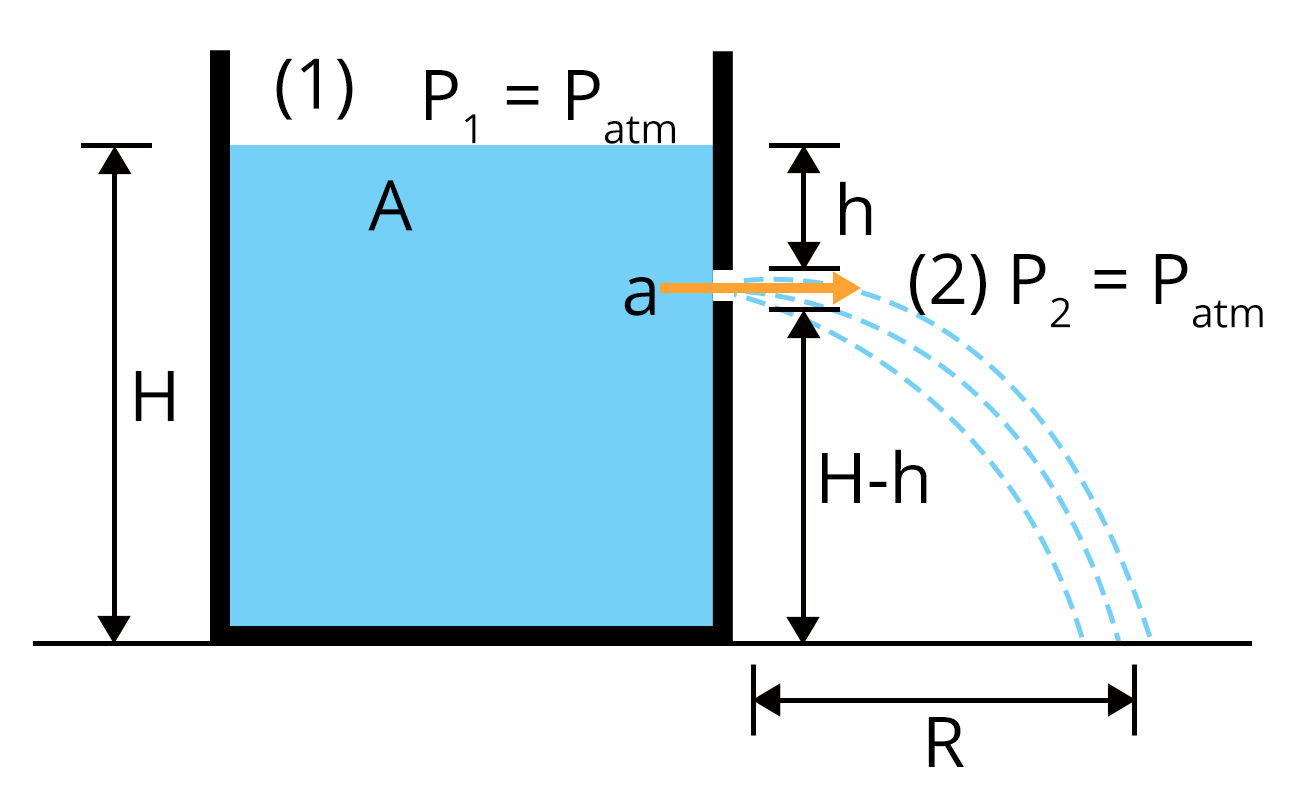14. The height of capillary rise inside a tube $h=\dfrac{2S\cos\theta}{\rho rg}$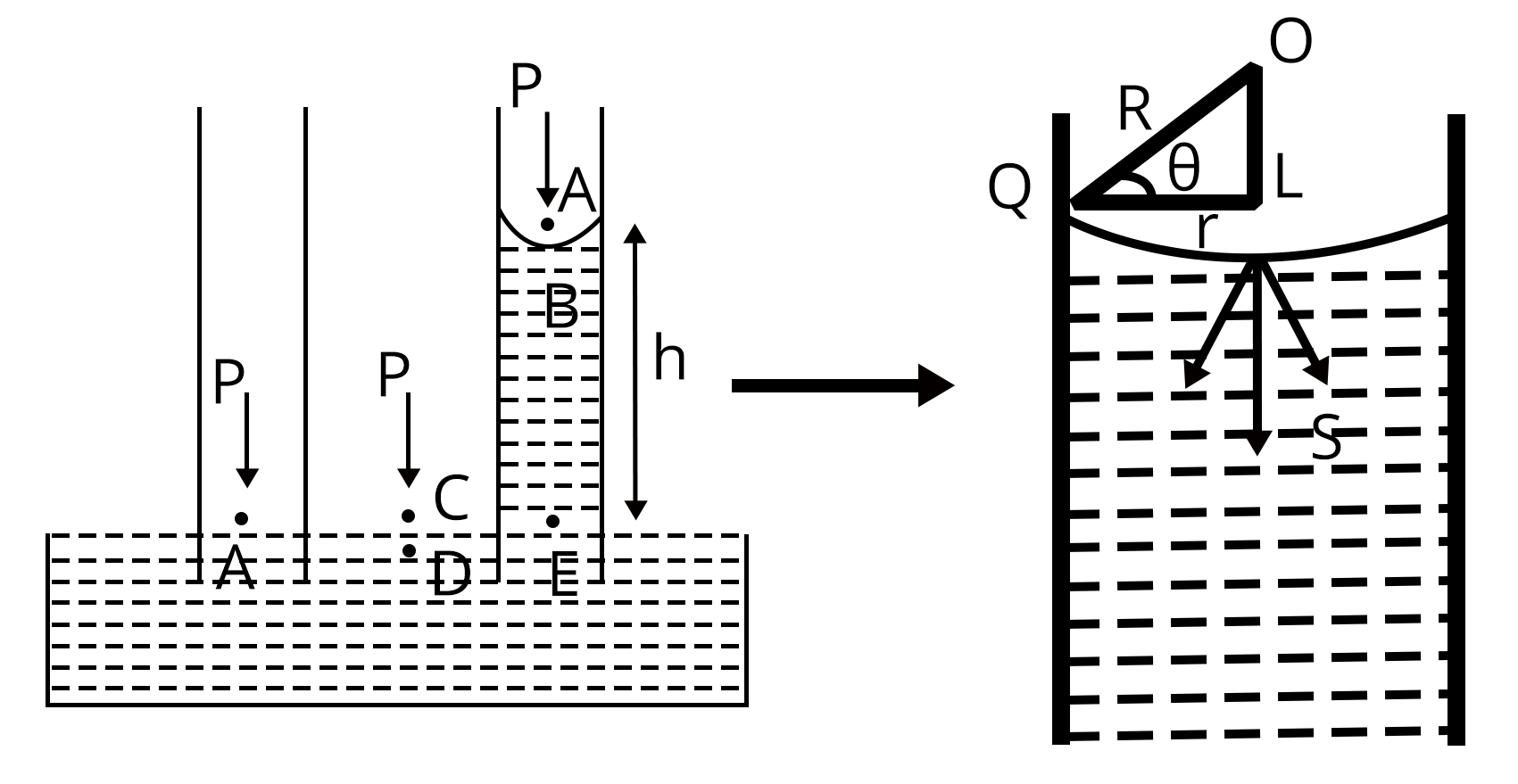### Solved Examples

1. A bomb of mass30 kg at rest explodes into two pieces of masses 18 kg and 12 kg. The velcoity of 18 kg mass is 6 m/s. The kinetic energy of the other mass is ________.

Sol:

The total momentum of the bomb before and after exploision is constant.

$0=m_1v_1+m_2v_2$

$0=18\times 6+12\times v_2$

$v_2=-9~ m/s$

The kinetic energy of the mass having 12 kg is given by,

$K=\dfrac{1}{2}mv^2$

$K=\dfrac{1}{2}\times 12\times 9^2$

$K=486~J$

Therefore, kinetic energy of the other mass is 486 J

Key Point: The total momentum is conserved in the case of explosion of a bomb.

2. The instantaneous angular position of a point on a rotating wheel is given by the equation, $\theta(t)=2t^3-6t^2$ . The torque on the wheel becomes zero at ______.

Sol:

We can find the equation of angular velocity from the angular position as given below,

$\omega=\dfrac{d\theta}{dt}$

$\omega=\dfrac{d}{dt}(2t^3-6t^2)$

$\omega=6t^2-12t$

The equation for angular acceleration of the point is obtained by,

$\alpha=\dfrac{d\omega}{dt}$

$\alpha=\dfrac{d}{dt}(6t^2-12t)$

$\alpha=12t-12$

The torque becomes zero when angular acceleration becomes zero

$\alpha=12t-12$

$0=12t-12$

$t=1~s$

Therefore, torque becomes zero at t =1 s.

Key Point: Differentiating angular velocity with respect to time gives angular acceleration.

### Previous Year Questions from JEE Advanced Paper

1. A wire which passes through the hole in a small bead, is bent in the form of quarter of a circle. The wire is fixed vertically on the ground as shown in the figure. The ball is released from near  the top of the wire and it slides along the wire without any friction. As the bead moves from A to B, the force it applies on the wire is  (JEE Advanced 2010)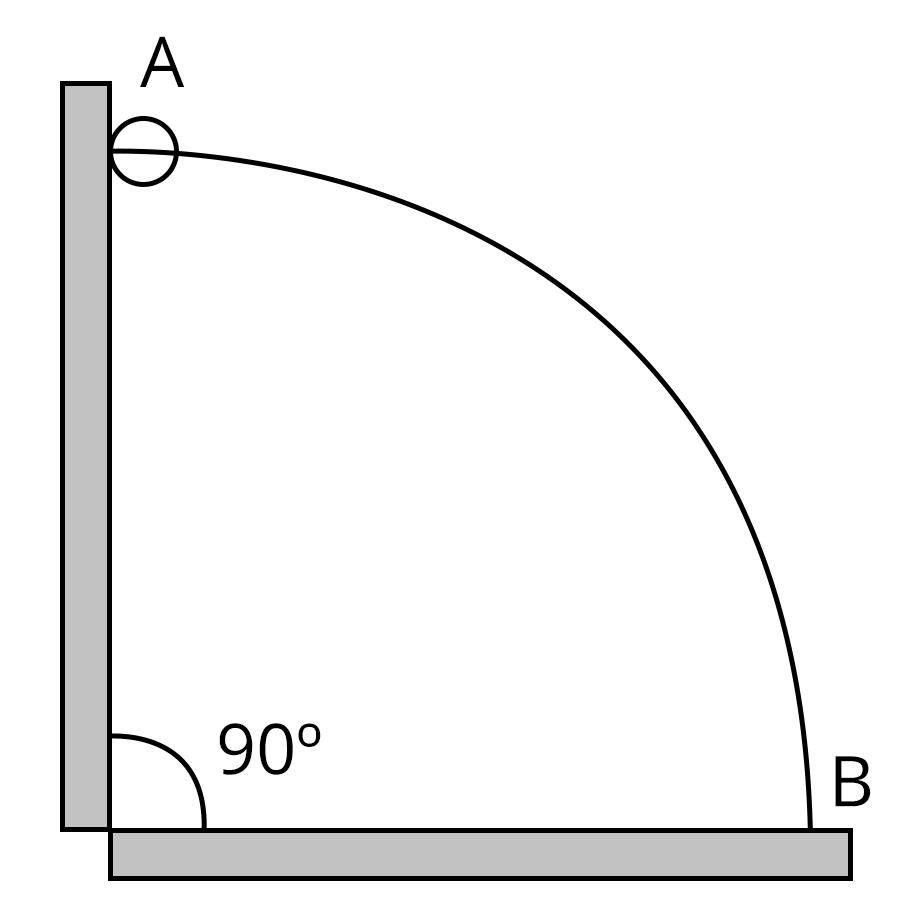Sol: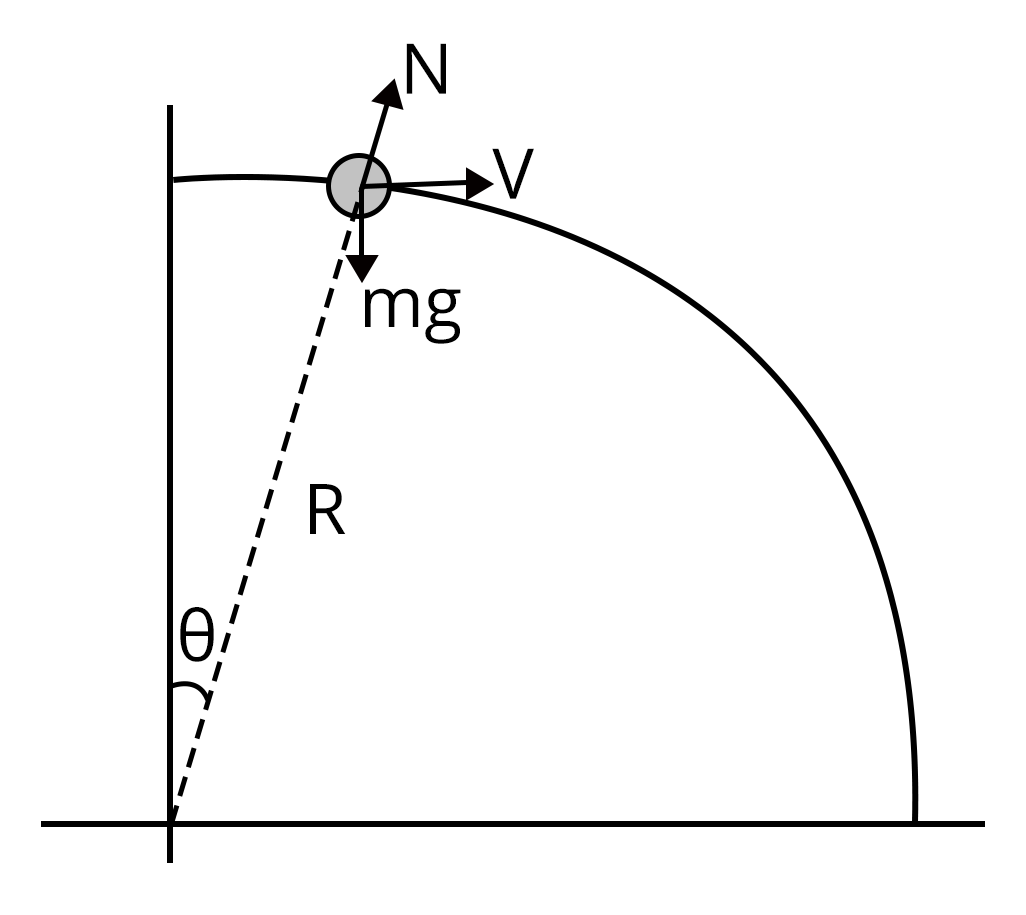Applying newton’s second law of motion equation,

$mg\cos\theta-\dfrac{mv^2}{R}=N$...(1)

Applying conservation of energy,

$\dfrac{1}{2}mv^2=mgR(1-\cos\theta)$...(2)

Using equation (1) and (2),

$N=mg(3\cos\theta-2)$

So, N is positive when

$0\lt \theta\lt \cos^{-1}\left(\dfrac{2}3{}\right)$

N is negative when

$\theta\gt \cos^{-1}\left(\dfrac{2}3{}\right)$

Therefore, force exerted  on the wire by the bead is radially inwards initially and radially outwards later. So, correct answer is option (D)

Key Point: For a body to move in a circular path, there must be a centripetal force acting on it.

2. Two identical uniform discs roll without slipping on two different surfaces,  AB and CD starting at A and C with linear speeds v1 and v2 respectively, and always remain in contact with surfaces. If they reach B and D with same linear speed v1= 3 m/s and v2= ______.(JEE Advanced 2015)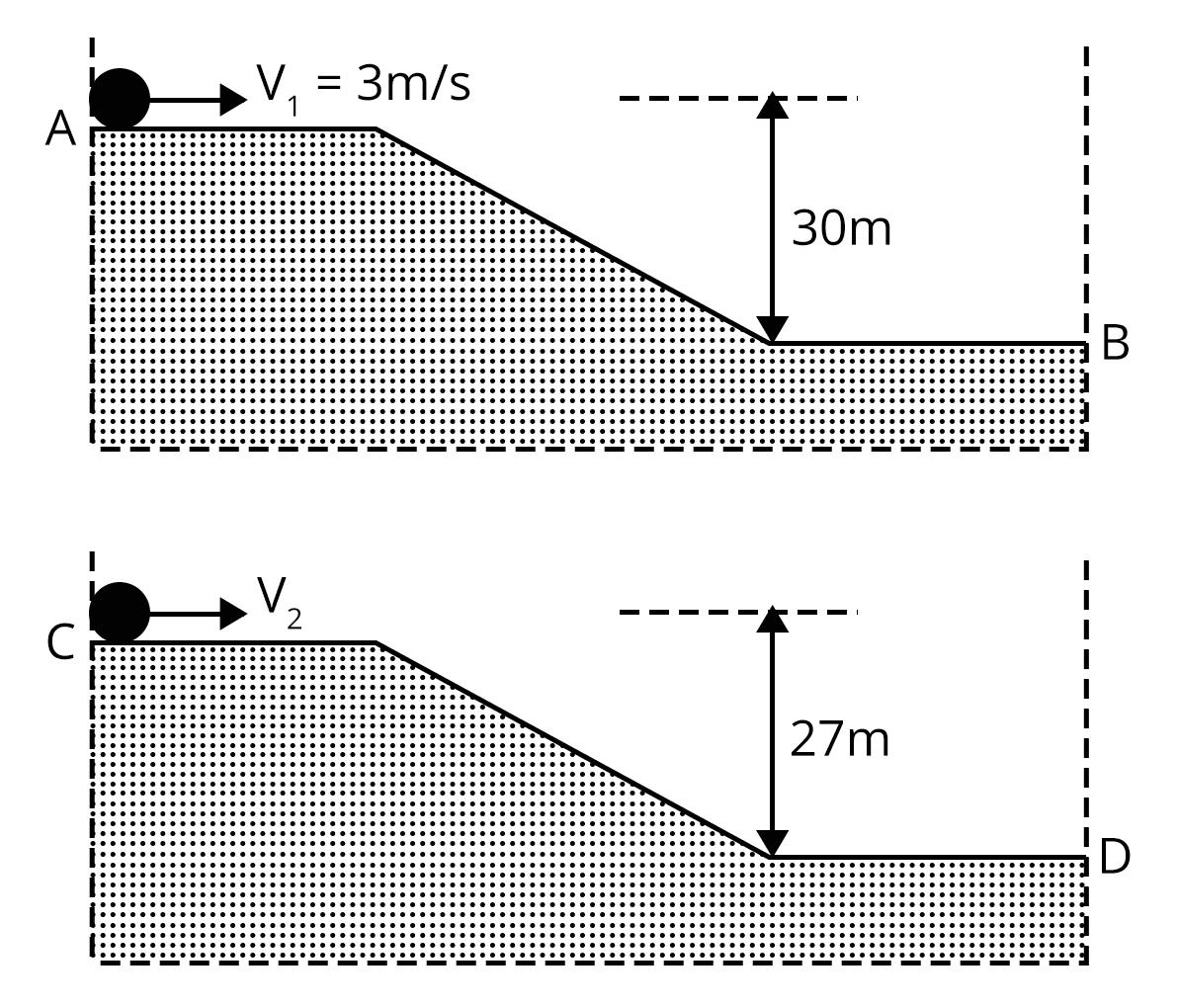Sol:

Both discs reach B and D with same speed. Hence, final kinetic energy is same for both disc.

Applying conservation of energy,

For first disc,

$\dfrac{1}{2}I\omega_1^2+mg\times30=K$...(1)

For second disc,

$\dfrac{1}{2}I\omega_2^2+mg\times27=K$.....(2)

Solving equation (1) and equation (2), we get,

$\dfrac{1}{2}\times\left(\dfrac{3}{2}mR^2\right)\times\left(\dfrac{3}{R}\right)^2+mg\times30=\dfrac{1}{2}\times\left(\dfrac{3}{2}mR^2\right)\times\left(\dfrac{v_2}{R}\right)^2+mg\times27$

$v_2=7~m/s$

Key Point: The total mechanical energy is constant if the work is done only by the conservative force.

### Practice Questions

1. A constant torque of 1000 Nm turns a wheel of moment of inertia 200 kgm2 about an axis through its centre. Its angular velocity after 3 seconds is  (Ans: 15 rad/s)

2. For a satellite moving in an orbit around the earth, the ratio of kinetic energy to the potential energy is (Ans: 1:2)

### Conclusion

In this article we have provided important information regarding the chapter mechanics such as important concepts, formulae, etc.. Students should work on more solved examples along with  previous year question papers for scoring good grades in the JEE advanced exams.

See MoreView all JEE Advanced Exam Dates
JEE Advanced 2023 exam date have been announced by the NTA. JEE Advanced 2023 will now be conducted on 4-June-2023, and the exam registration closes on 7-May-2023. You can check the complete schedule on our site. Furthermore, you can check JEE Advanced 2023 dates for application, admit card, exam, answer key, result, counselling, etc along with other relevant information.
See More
View all JEE Advanced Exam DatesApplication Form
Eligibility Criteria
Reservation Policy
IIT Guwahati has announced the JEE Advanced 2023 application form release date on the official website https://jeeadv.ac.in/. JEE Advanced 2023 Application Form is available on the official website for online registration. Besides JEE Advanced 2023 application form release date, learn about the application process, steps to fill the form, how to submit, exam date sheet etc online. Check our website for more details.View JEE Advanced Syllabus in Detail
It is crucial for the the engineering aspirants to know and download the JEE Advanced 2023 syllabus PDF for Maths, Physics and Chemistry. Check JEE Advanced 2023 syllabus here along with the best books and strategies to prepare for the entrance exam. Download the JEE Advanced 2023 syllabus consolidated as per the latest NTA guidelines from Vedantu for free.
See More
View JEE Advanced Syllabus in Detail## JEE Advanced 2023 Study Material

View all study material for JEE Advanced
JEE Advanced 2023 Study Materials: Strengthen your fundamentals with exhaustive JEE Advanced Study Materials. It covers the entire JEE Advanced syllabus, DPP, PYP with ample objective and subjective solved problems. Free download of JEE Advanced study material for Physics, Chemistry and Maths are available on our website so that students can gear up their preparation for JEE Advanced exam 2023 with Vedantu right on time.
See More
All
Chemistry
Maths
Physics
See Allsee all
See MoreView all JEE Advanced Important Books
In order to prepare for JEE Advanced 2023, candidates should know the list of important books i.e. RD Sharma Solutions, NCERT Solutions, HC Verma books and RS Aggarwal Solutions. They will find the high quality readymade solutions of these books on Vedantu. These books will help them in order to prepare well for the JEE Advanced 2023 exam so that they can grab the top rank in the all India entrance exam.
See More
Maths
NCERT Book for Class 12 Maths
Physics
NCERT Book for Class 12 Physics
Chemistry
NCERT Book for Class 12 Chemistry
Physics
H. C. Verma Solutions
Maths
R. D. Sharma Solutions
Maths
R.S. Aggarwal Solutions
See AllView all mock tests
See MoreIIT Bombay is responsible for the release of the JEE Advanced 2023 cut off score. The qualifying percentile score might remain the same for different categories. According to the latest trends, the expected cut off mark for JEE Advanced 2023 is 50% for general category candidates, 45% for physically challenged candidates, and 40% for candidates from reserved categories. For the general category, JEE Advanced qualifying marks for 2021 ranged from 17.50%, while for OBC/SC/ST categories, they ranged from 15.75% for OBC, 8.75% for SC and 8.75% for ST category.
See MoreSee More
Rank List
Counselling
Cutoff
JEE Advanced 2022 State Wise Rank List - The respective state authorities started releasing the JEE Advanced 2022 state merit lists for admission to Engineering course. Check JEE Advanced 2022 state-wise rank list for the year 2022 here.View all JEE Advanced 2023 Top Colleges
Want to know which Engineering colleges in India accept the JEE Advanced 2023 scores for admission to Engineering? Find the list of Engineering colleges accepting JEE Advanced scores in India, compiled by Vedantu. There are 1622 Colleges that are accepting JEE Advanced. Also find more details on Fees, Ranking, Admission, and Placement.
See More## FAQs on JEE Important Chapter - Mechanics

FAQ

1. What is the weightage of mechanics in JEE Advanced?

Nearly 25% questions are asked in the JEE Advanced exam from this chapter. Mechanics is one of the most important topics and fundamental topic in JEE Advanced.

2. What is the difficulty level of Mechanics in JEE Advanced exam?

Theory of Mechanics is a vast topic and one of the fundamentals of physics required for JEE advanced. The mixed concepts from different types of mechanics topics  can also asked by relating to other topics of JEE Asvanced. Students who are good in mechanics can score good marks in JEE advanced.  Overall, mechanics is a moderate difficult which becomes easier with proper practise and effort. It is better if yo keep mechanics of structure pdf readily available for reference.

3. Are previous year's questions Important for JEE Advanced?

Yes, previous year's JEE Advanced questions are important. Once the student is finished learning concepts and solving problems, they must go through the previous year's question papers the last 20 years to understand the test pattern and frequent questions asked in the exam.04 Jun 20232 days remainingJEE (Advanced) 2023 Examination Paper 1
04 Jun 20231 days remainingJEE (Advanced) 2023 Examination Paper 2
04 Jun 20232 days remainingOnline display of provisional answer keys
11 Jun 20238 days remainingArchitecture Aptitude Test (AAT) 2023
21 Jun 202318 days remainingDeclaration of results of AAT 2023
24 Jun 202322 days remaining## Notice board

JEE News
JEE BlogsTrending pages